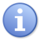# Defining Cartesian Groups

## Introduction

Cartesian groups are enhancements of groups. Like groups, they are a collection of axes that can be moved together. In addition, they have a predefined Cartesian model for defining the roll of each axis in the Cartesian space.

## Creating a Cartesian Group

Cartesian groups are defined similar to ordinary groups, either in configuration file or as a terminal input. The only difference is that at the end the type of the group is defined by: model=1 of <point type>, where <point type> is a string that defines the actual Cartesian model, which can be one of the following:

 Mnemonic Constant used in CASTPOINT Numerical Value used in CASTPOINT Function String Explanation NO_ROBOT 0 NONE List-of-coordinates, uninitialized generic points TYPE_X 1 X X coordinate only TYPE_XY 2 XY X Y table TYPE_XYZ 3 XYZ X Y Z TYPE_XYZU 4 XYZU TYPE_XYZUV 5 XYZUV TYPE_XYZUVW 6 XYZUVW TYPE_XYR 7 XYR X Y Roll TYPE_XYRZ 8 XYRZ TYPE_XYRZU 9 XYRZU TYPE_XYRZUV 10 XYRZUV TYPE_XYZR 11 XYZR X Y Z Roll TYPE_XYZRU 12 XYZRU TYPE_XYZRUV 13 XYZRUV TYPE_XYZURV 14 XYZURV TYPE_XYZUR 15 XYZUR TYPE_XYZRPU 16 XYZRPU TYPE_XYZRP 17 XYZRP X Y Z Roll Pitch TYPE_XYRURV 18 XYZRURV TYPE_XYRUR 19 XYRUR TYPE_XYRP 20 XYRP X Y Roll Pitch TYPE_XYRPU 21 XYRPU TYPE_XYRPUV 22 XYRPUV TYPE_XYRPUQ 23 XYRPUQ TYPE_XYZYPR 24 XYZYPR X Y Z Yaw Pitch Roll TYPE_XYZPR 36 User defined point TYPE_XYZA 37 XYZA X Y Z A-angle TYPE_XYZAB 38 XYZAB X Y Z A-angle B-angle TYPE_YPR 50 YPR Yaw Pitch Roll TYPE_PR 51 PR Pitch Roll TYPE_R 52 R Roll

For example, the following defines a Cartesian group called GXYZ:

```common shared GXYZ   as group Axnm = a1 Axnm = a2 Axnm = a3 model=1  of xyz
```

## Usage

Cartesian groups implement robot models with 1-1 Inverse and Direct kinematics functions. For example, an XY Cartesian group is defined by following mapping:

J1 ←→ X
J2 ←→ Y

This means that coordinates of <group.setpoint and <group>.pcmd will have equal values.

## Translation vs. Rotation

The greatest difference between ordinary groups and Cartesian groups is the separation of translational and rotational movements. This further means that axis type must mach the Cartesian model:

For example, in XYR group (X, Y and Roll) axistype must be set as:

```Common Shared GXYR as Group AxisName=A1 AxisName=A2 AxisName=A3 model = 1 of XYR
...
a1.axistype = 1
a2.axistype = 1
a3.axistype = 1
ConfigGroup GXYR
```

If axis type is not properly set, ConfigGroup command will return an error. When such a group is properly set it allows setting physical kinematics values of velocity, acceleration and jerk separated into translational and rotational parts in the same way it is done in robot models:

```MoveS GXYR #{100,100,90} VelTran=100 VelRot=20
```

This will move the GXYR to the target point (X=100mm, Y=100mm, R = 90deg) with 100 mm/sec or 20 deg/sec, depending on which values dominates (takes more time).

## Motion

The following motion interpolation types are defined in Cartesian groups:

• Move - joint interpolations, used Vcruise, Vfinal in non-physical user units.
• MoveS - "straight-line" interpolation with separated kinematics parameters (Vtra/Vrot, Atran/Arot, Jtran/Jrot) defined in physical units for rotation and translation.
• Circle - circular/helycal interpolation in XYZ space with proportional change of additional rotation axes with separated kinematics parameters (Vtra/Vrot, Atran/Arot, Jtran/Jrot) defined in physical units for rotation and translation.NOTE There is no difference in the path of Move and Moves interpolation, except for how the time derivatives are determined.